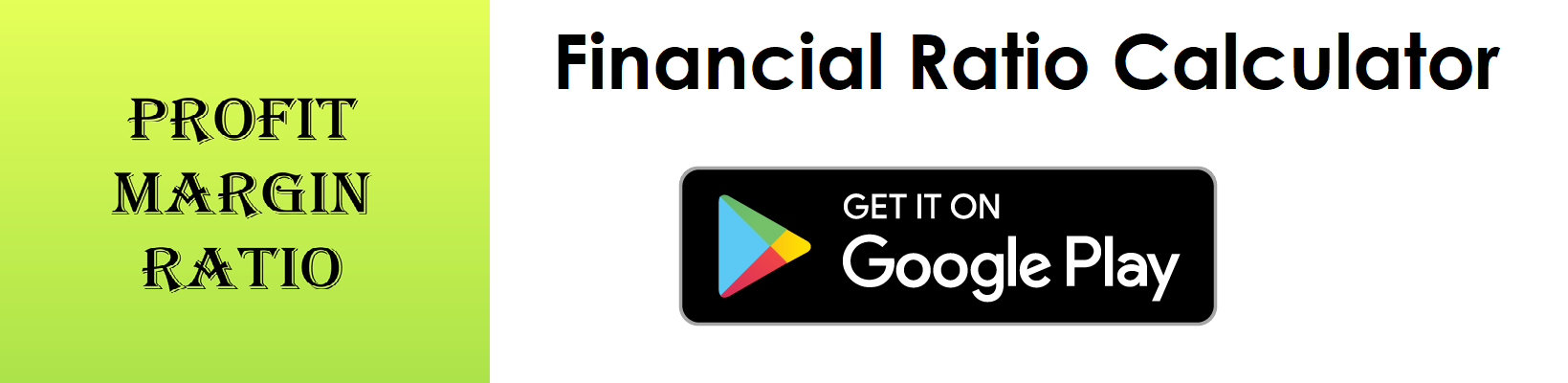# Asset Turnover Ratio Calculator

Key in the Net Sales and Total Assets Values to the respective fields given below and then click Calculate to get the desired result.

##### Asset Turnover Ratio Calculator - Glossary:
Using our asset turnover ratio calculator, helps to compare, measure, understand the overall health of the business.

##### Asset Turnover Ratio:
Shows the proportion of Net Sales to its Average Total Assets. In simple words, it measures the efficiency of a company’s ability to use its asset to generate sales.

##### How to calculate?
Formula: Asset Turnover Ratio = Net Sales/Average Total Assets.
The values are commonly stated against total assets in the balance and net sales in the income statement.
Note:
Average Total Assets = (Beginning Total Assets + Ending Total Assets)/2.

##### Net Sales:
Total sales less returns and bad debts.

##### Total Assets:
A balance sheet component - Total assets are the sum of all current and noncurrent assets that a company owns.

##### Average Total Assets:
An average of total assets value at the beginning of the year and total assets value at the end of the year.

##### Example:
Asset turnover ratio for a company with a net income of \$500,000 and average total assets of \$775,000 is 0.65:1. It means that for every dollar in assets, a company generates \$0.65 in sales.

# Download our Mobile Apps - today.